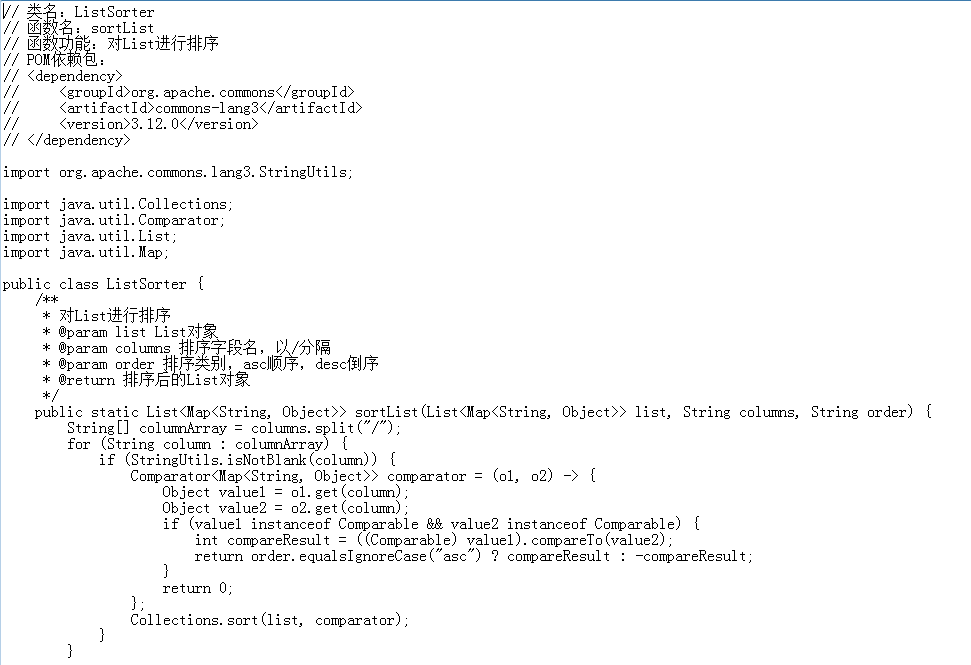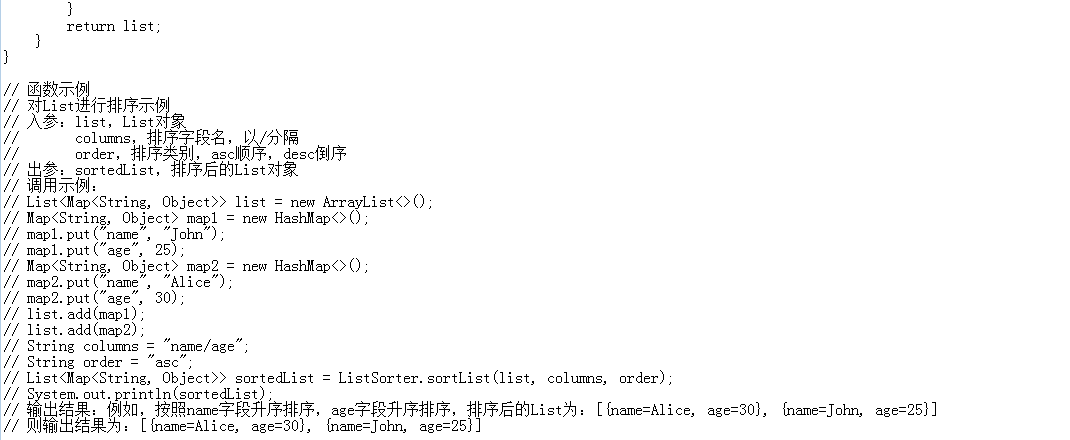# 4招搞定Java List排序

08/16 17:19

1.使用 Comparable 进行排序；

2.使用 Comparator 进行排序;

3. JDK 8 以上的环境，可以使用 Stream 流进行排排序；

4. JDK8之后特别是lambda表达式的盛行，而且Collections的sort方法其实是调用了List接口自己的sort方法;所以可以使用List接口自己的sort方法排序。

1. 人工编写：使用Comparator排序

public static void sort(List<Map<String, Object>> list, String columns, String order) {

String[] arrCols = columns.split(REGEX);

Stream<Map<String, Object>> stream = list.stream();

Comparator<Map<String, Object>> comparator = getMapComparator(list, arrCols, order);

List<Map<String, Object>> updateList =    stream.sorted(comparator).collect(Collectors.toList());

list.clear();

}

private static Comparator<Map<String, Object>> getMapComparator(List<Map<String, Object>> list, String[] arrCols, String order) {

Comparator<Map<String, Object>> comparator;

if (list.get(0).get(arrCols) instanceof Integer) {

comparator = Comparator.comparingInt((Map<String, Object> map) -> Integer.valueOf(map.get(arrCols).toString()));

} else if (list.get(0).get(arrCols) instanceof Long) {

comparator = Comparator.comparingLong((Map<String, Object> map) -> Long.valueOf(map.get(arrCols).toString()));

} else if (list.get(0).get(arrCols) instanceof Double) {

comparator = Comparator.comparingDouble((Map<String, Object> map) -> Double.valueOf(map.get(arrCols).toString()));

} else {

comparator = Comparator.comparing((Map<String, Object> map) -> String.valueOf(map.get(arrCols)));

}

//降序

if (order.equals(ORDER_DESC)) {

comparator = comparator.reversed();

}

//排除第一个

for (int i = 1; i < arrCols.length; i++) {

int f = i;

if (list.get(0).get(arrCols[f]) instanceof Integer) {

comparator = comparator.thenComparingInt((Map<String, Object> map) -> Integer.valueOf(map.get(arrCols[f]).toString()));

} else if (list.get(0).get(arrCols[f]) instanceof Long) {

comparator = comparator.thenComparingLong((Map<String, Object> map) -> Long.valueOf(map.get(arrCols[f]).toString()));

} else if (list.get(0).get(arrCols[f]) instanceof Double) {

comparator = comparator.thenComparingDouble((Map<String, Object> map) -> Double.valueOf(map.get(arrCols[f]).toString()));

} else {

comparator = comparator.thenComparing((Map<String, Object> map) -> String.valueOf(map.get(arrCols[f])));

}

//如果是降序每次都设置降序

if (order.equals("desc")) {

comparator = comparator.reversed();

}

}

return comparator;

}

1. FuncGPT(慧函数)函数AI 生成器：使用Collection.sort排序

Collection.sort()用来对集合进行排序, 其中sort和冒泡排序差不多，sort方法是不断的遍历List，比较List的任意相邻两个元素，看看这两个相邻的元素是否发生交换。如果List中任意两个元素都不会发生交换，说明排序结束。1. 总结FuncGPT（慧函数）免费开放中，点击链接http://suo.im/aREPi下载安装。0 评论
0 收藏
0